# Balance

The rod are 1.9 m long hanging weights 4 kg and 1 kg on ends. Where are centre of rod (distance from weight 4 kg) to be in balance?

Correct result:

x =  0.38 m

#### Solution: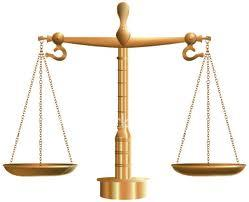We would be very happy if you find an error in the example, spelling mistakes, or inaccuracies, and please send it to us. We thank you!Tips to related online calculators
Looking for help with calculating arithmetic mean?
Looking for a statistical calculator?
Do you have a linear equation or system of equations and looking for its solution? Or do you have quadratic equation?
Do you want to convert length units?

#### You need to know the following knowledge to solve this word math problem:

We encourage you to watch this tutorial video on this math problem:

## Next similar math problems:

• Heating plant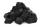Workers dump imported coke at heating plant. On the first day used half of the amount, the second day three quarters the rest and on the third day left 120 tons. How much coke they dump at heating plant?
• Simple equationSolve for x: 3(x + 2) = x - 18
• AverageIf the average(arithmetic mean) of three numbers x,y,z is 50. What is the average of there numbers (3x +10), (3y +10), (3z+10) ?
• Average height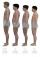In a class are 34 students. The average height of students is 165 cm. What will be the average height of students in the classroom when two pupils tall 176 cm and 170 cm moved from this school/class?
• Fifth of the numberThe fifth of the number is by 24 less than that number. What is the number?
• Meanif the mean of the set of data 5, 17, 19, 14, 15, 17, 7, 11, 16, 19, 5, 5, 10, 8, 13, 14, 4, 2, 17, 11, x is -91.74, what is the value of x?
• Bakery and flour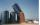The bakery tray for flour was filled to 3/4 volume. After removing 875 kg of flour, it was filled to only 2/5 of the volume. How many tons of flour is in the full tray?
• 75th percentile (quartille Q3)Find 75th percentile for 30,42,42,46,46,46,50,50,54
• Unknown numberIdentify unknown number which 1/5 is 40 greater than one tenth of that number.
• AverageThe arithmetic mean of the two numbers is 71.7. One number is 5. Calculate the second number.
• Median and modusRadka made 50 throws with a dice. The table saw fit individual dice's wall frequency: Wall Number: 1 2 3 4 5 6 frequency: 8 7 5 11 6 13 Calculate the modus and median of the wall numbers that Radka fell.
• EquationSolve the equation: 1/2-2/8 = 1/10; Write the result as a decimal number.
• CandyPeter had a sachet of candy. He wanted to share with his friends. If he gave them 30 candies, he would have 62 candies. If he gave them 40 candies, he would miss 8 candies. How many friends did Peter have?
• Trees avgThe worker planted 96 trees on Monday, 120 on Tuesday and 61 trees on Wednesday. How many trees did he plant on Thursday if he averaged 105 trees per day?
• Three friends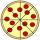Divide 570 euros to three friends so that first will get 50 euros less than the second and third twice more than the first. How many euros will get everyone?
• MeatHalf a kilogram of pork and three-quarters of a kilogram of beef cost total 5.1 USD. One quarter of a kilogram of beef and 1 kilogram of pork cost 4.9 USD. Determine the price of 1 kg of each type of meat.
• CandyThe price for 1kg of more expensive candy is 125 CZK. The price for 1kg of cheaper candy is 100 CZK. We make two different blends of candy. And now. The second blend contains 2kg of more expensive candy and several kg of cheaper candy. The price per 1 kg